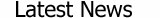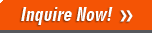Weight Conversion

=

Length Conversion

=

Cubage Conversion

=

Pressure Conversion

 MPakPaPapsi0℃mmHg0℃inHg15℃mmH2O15℃inH2Okgf/cm2atmbarmbarTorr = MPakPaPapsi0℃mmHg0℃inHg15℃mmH2O15℃inH2Okgf/cm2atmbarmbarTorr

 △ Enter the conversion value △ Select the pressure unit of conversion value △ Here shows the conversion or the result of conversion △ Select the pressure unit to convert

1、Other system of units of measurement→Metric:
Fill in the non-standard number of units of measurement in the left side of equation of text input box, such as “1”. Select unit in the left side of equation of non-standard units of measurement drop down menu, such as “Pound”. Select unit in the right side of equation of metric units of measurement drop down menu, such as “KG”. Click “Other→Metric” button, “0.4536”KG is gotten in the right side of equation of text input box.

2、Metric→Other system of units of measurement:
Fill in the metric number of units of measurement in the right side of equation of text input box, such as “1”. Select unit in the right side of equation of metric units of measurement drop down menu, such as “KG” . Select unit in the left side of equation of non-standard units of measurement drop down menu, such as “Pound”. Click “Metric→Other”button, “2.205” pound is gotten in the left side of equation of text input box.

3、Other conversion between units of measurement:
For example, calculating the relationship of "Jin" and "pound". Fill in “1” in the left side of equation of text input box. Select “Jin” in the left side of drop down menu. Click “Other→Metric” button, select “Pound” in the left side of equation of drop down menu. Click “Metric→Other”button, 1 Jin equals to “1.102” pound.

4、Conversion between metric units:
For example, calculating the relationship of "KG” and “G”. Fill in “1” in the right side of equation of text input box..Select “KG” in the right side of drop down menu. Select “Jin” in the left side of drop down menu (or other valid units).Click “Metric→Other”button, it is “ 2” Jin, then select “G” in the right side of droop down menu. Click “Other→Metric”, 1 KG equals to “1000” G.

5、The converter and Netscape browser are not compatible, please forgive.

Other important product is small pellet machines.

Product List

Special Steel﻿